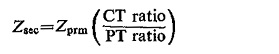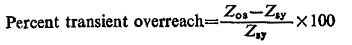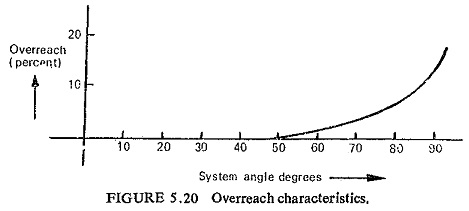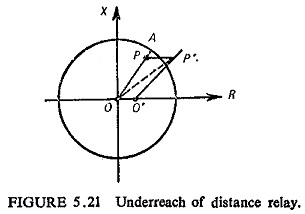## Reach of Distance Relay:

A distance relay is set to operate up to a particular value of impedance; for an impedance greater than this set value the relay should not operate. This impedance, or the corresponding distance is known as the Reach of Distance Relay.

To convert primary impedance (impedance of the line referred to the line voltage and current) to a secondary value (line impedance referred to the relay side) for use in adjusting a distance relay the following relation is used:where the CT ratio is the ratio of the HV phase current to the relay phase current, and the PT ratio is the ratio of the HV phase-to-phase voltage to the relay phase-to-phase voltage all under balanced conditions.

The tendency of a Reach of Distance Relay to operate at impedance larger than its setting value is known as overreach and similarly the tendency to restrain at the set value of impedance or impedances lower than the set value is known as underreach.

An important reason for overreach is the presence of d.c. offset in the fault current wave, as the offset current has a higher peak value than that of a symmetrical wave for which the relay is set.

The transient overreach is defined as:Where

Zos = the maximum impedance for which the relay will operate with an offset current wave, for a given adjustment

Zsy = the maximum impedance for which the relay will operate for symmetrical currents for the same adjustment as for Zos.

The transient overreach increases as the system angle tan-1 X/R increases. Figure (5.20) shows the variation of overreach with system angle.A distance relay may underreach because of the introduction of fault resistance as illustrated in Fig. (5.21). Relay at O is set for protection up to P. Now if a fault at P occurs such that fault resistance (PP’) is high and by adding this resistance the impedance seen by the relay is OP’ such that P’ lies outside the operating region of the relay, then the relay does not operate.Occasionally a fault may occur in the zone 1 and the relay may begin to operate. If the fault impedance now increases due to the arc resistance the total impedance seen by the relay will be the sum of the line impedance up to the fault and the arc impedance. This sum may be more than the impedance setting of the relay in which case the 1st stage operation will stop and the fault will be cleared as if it is located in the second or third zone. In order to prevent this impedance relays are locked once they begin to operate on the basis of the true impedance up to the fault. Any increase in impedance due to are will not affect the relay once it is locked.

Scroll to Top